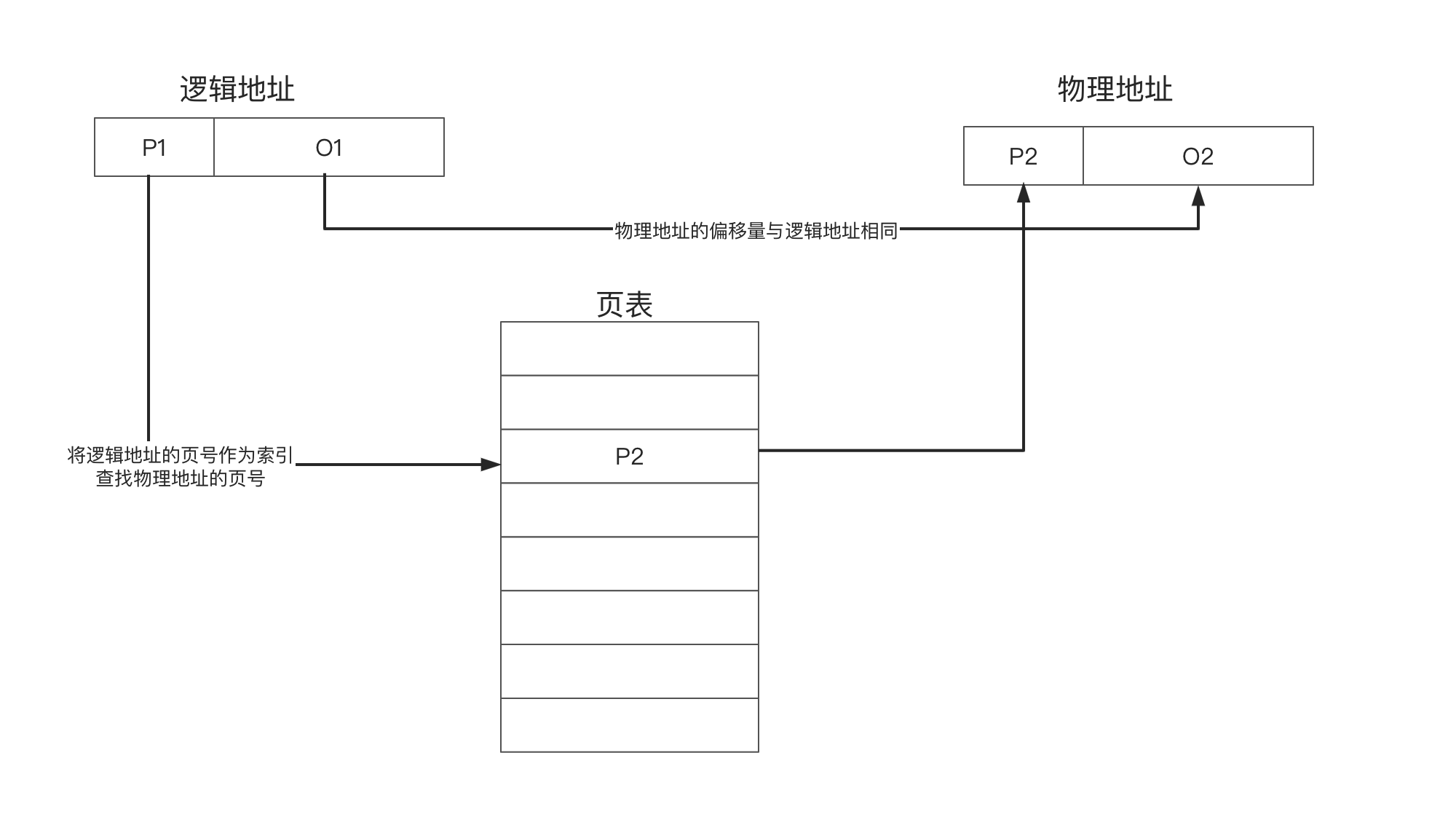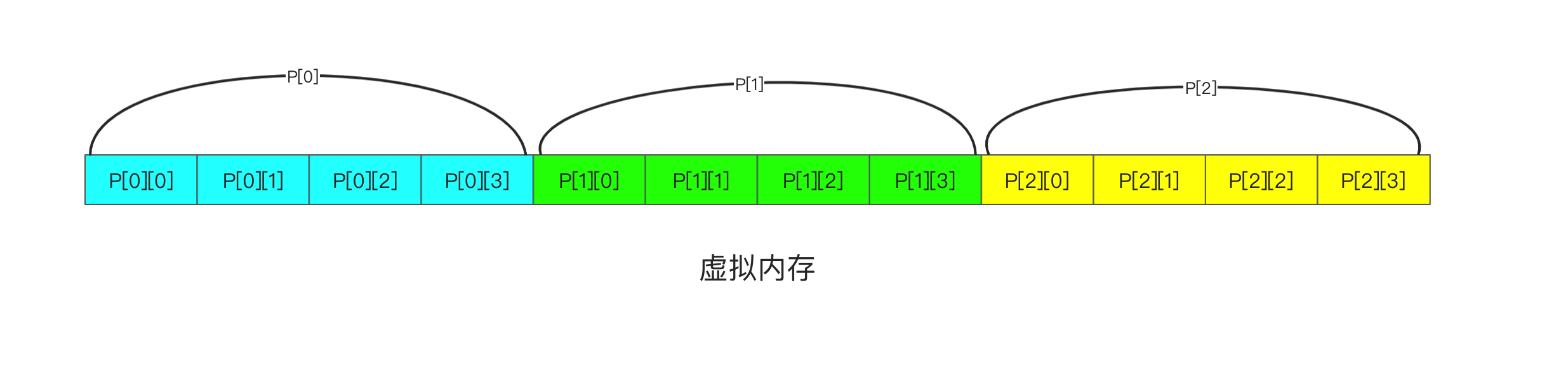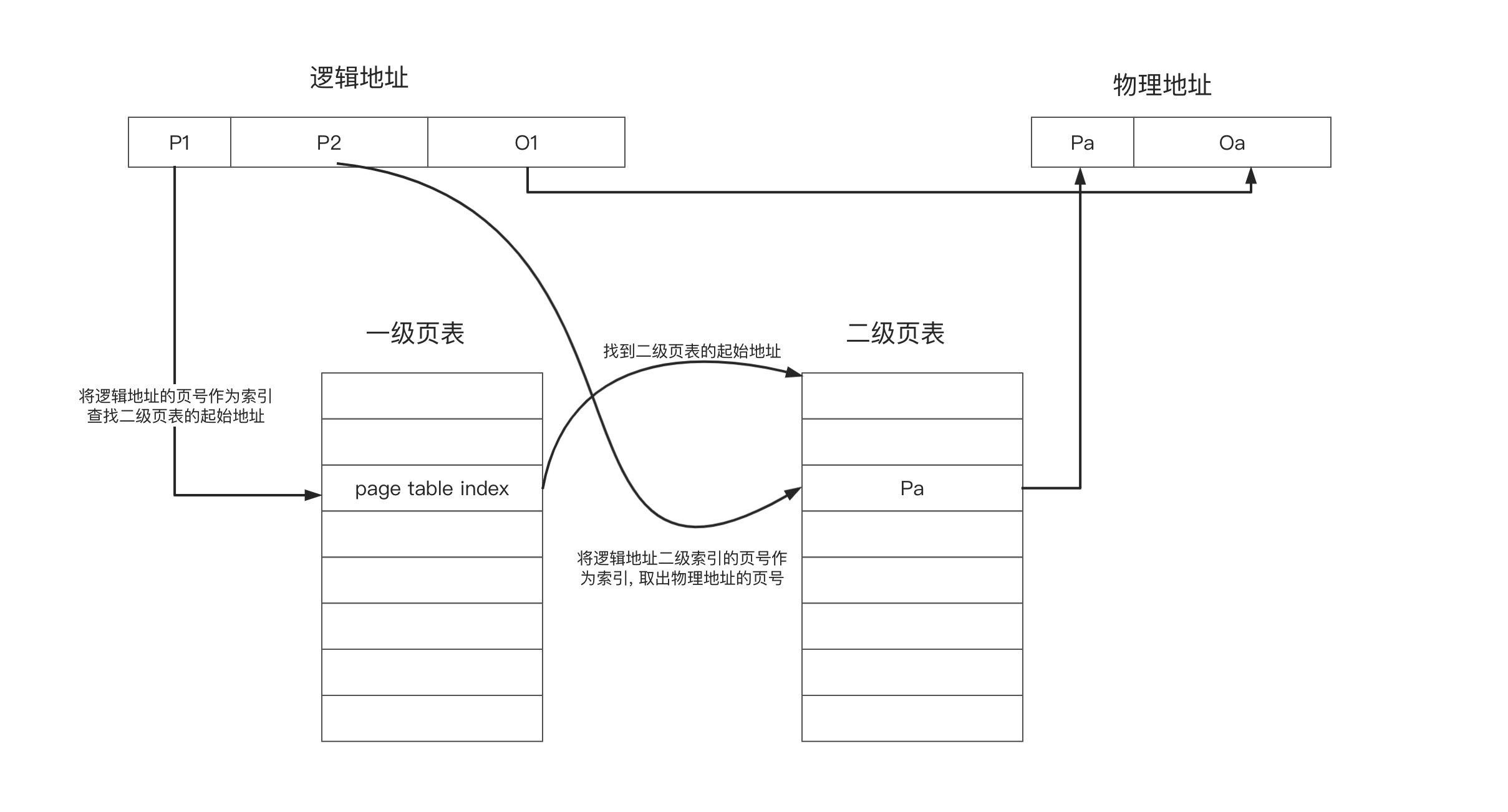# 概述

• 页号: 第几页
• 偏移量: 当前页的第几个字节

# 页表• 存在位: 当前也是否加载到内存中了. 若没有加载需要操作系统进行加载
• 修改位: 当前页在内存中是否被修改过. 若修改过, 则回收物理内存时需要将其写会硬盘
• 内核权限: 当前页是否需要内核模式才能访问
• 是否可读位
• 是否可写位
• 是否可执行
• 等等

## 问题与解决方案

### 时间问题

1. 查找页表找到对应的物理地址
2. 访问物理地址

#### 解决方案

1. 查看TLB中是否存在缓存, 若存在直接获取
2. TLB中不存在, 从内存页表中获取并放入TLB

TLB存在的前提, 是程序的访问具有局部性. 终于, 又是程序的局部性救了我们.

### 空间问题

4G / 4kb = (2^32) / (2^12) = 2^20 = 1mb

#### 解决方案1. 一级页表的索引
2. 二级页表的索引

# 程序的局部性

int main() {
int i, j;
int arr;
// 第一种方式
for(i = 0; i < 1024; i++){
for(j = 0; j < 1024; j++){
global_arr[i][j] = 0;
}
}
// 第二种方式
for(j = 0; j < 1024; j++){
for(i = 0; i < 1024; i++){
global_arr[i][j] = 0;
}
}
}

1. TLB容量不足, 新的缓存会淘汰旧的缓存, 频繁访问不同的页会造成更多的缓存失效
2. 若内存容量不足, 写入新的页会淘汰旧的页, 频繁访问不同的页会导致更多内存的换入换出.

## 口说无凭

#include <stdio.h>
#include <sys/resource.h>

const int M = 1024;
// 增加列的大小, 以使得效果明显. 10mb
const int N = 1024*10;
// 因为限制了栈的大小, 故将变量提升为全局, 放到堆中
int global_arr[1024*10];

int main() {
int i, j;
// 第一种方式
for(i = 0; i < M; i++){
for(j = 0; j < N; j++){
global_arr[i][j] = 0;
}
}
// 第二种方式
//    for(j = 0; j < N; j++){
//        for(i = 0; i < M; i++){
//            global_arr[i][j] = 0;
//        }
//    }

struct rusage usage;
getrusage(RUSAGE_SELF, &usage);
printf("页回收次数: %ld\n", usage.ru_minflt);
printf("缺页中断的次数: %ld\n", usage.ru_majflt);
}

docker run -it -m 6m --memory-swap -1 debian bash0 评论

0